• +91 9971497814
• info@interviewmaterial.com

# RD Chapter 23- The Straight Lines Ex-23.15 Interview Questions Answers

### Related Subjects

Question 1 : Find the distance of the point (4, 5) from the straight line 3x – 5y + 7 = 0.

Given:

The line: 3x – 5y+ 7 = 0

Comparing ax + by + c= 0 and 3x − 5y + 7 = 0, we get:

a = 3, b= − 5 and c = 7

So, the distance ofthe point (4, 5) from the straight line 3x − 5y + 7 = 0 is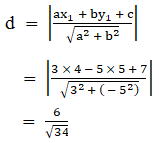The required distanceis 6/√34

Question 2 : Find the perpendicular distance of the line joining the points (cos θ, sin θ) and (cos ϕ, sin ϕ) from the origin.

Given:

The points (cos θ, sinθ) and (cos ϕ, sin ϕ) from the origin.

The equation of theline joining the points (cos θ, sin θ) and (cos ϕ, sin ϕ)is given below: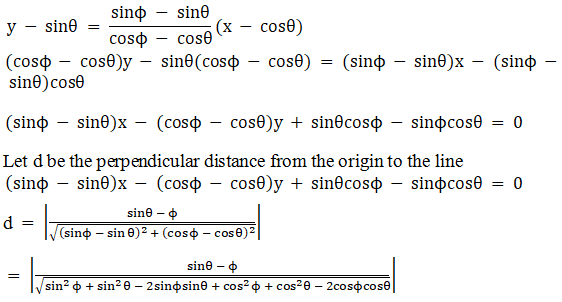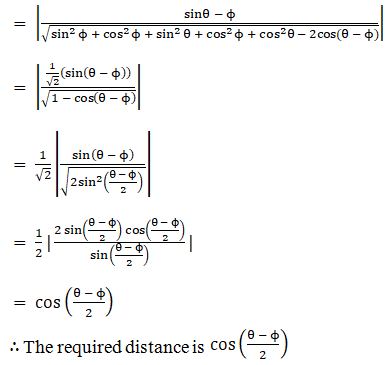Question 3 : Find the length of the perpendicular from the origin to the straight line joining the two points whose coordinates are (a cos α, a sin α) and (a cos β, a sin β).

Given:

Coordinates are (acos α, a sin α) and (a cos β, a sin β).

Equation of the linepassing through (a cos α, a sin α) and (a cos β, a sin β) is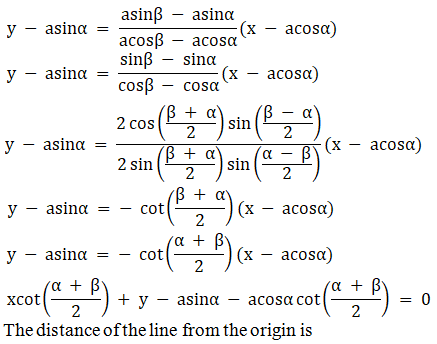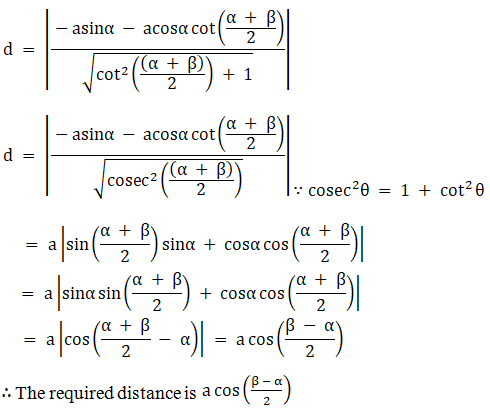Question 4 : Show that the perpendicular let fall from any point on the straight line 2x + 11y – 5 = 0 upon the two straight lines 24x + 7y = 20 and 4x – 3y – 2 = 0 are equal to each other.

Given:

The lines 24x + 7y =20 and 4x – 3y – 2 = 0

Let us assume, P(a, b)be any point on 2x + 11y − 5 = 0

So,

2a + 11b − 5= 0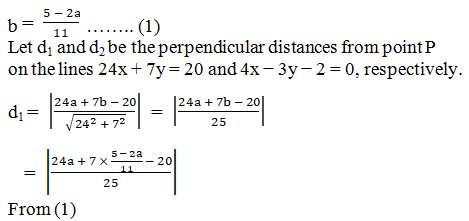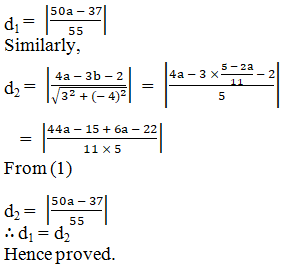Question 5 : Find the distance of the point of intersection of the lines 2x + 3y = 21 and 3x – 4y + 11 = 0 from the line 8x + 6y + 5 = 0.

Given:

The lines 2x + 3y = 21and 3x – 4y + 11 = 0

Solving the lines 2x +3y = 21 and 3x − 4y + 11 = 0 we get: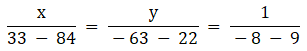x = 3, y = 5

So, the point ofintersection of 2x + 3y = 21 and 3x − 4y + 11 = 0 is (3, 5).

Now, the perpendiculardistance d of the line 8x + 6y + 5 = 0 from the point (3, 5) is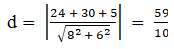The distance is59/10.

Todays Deals### RD Chapter 23- The Straight Lines Ex-23.15 Contributorskrishan

Name:
Email:

# Latest News# 9000 interview questions in different categories